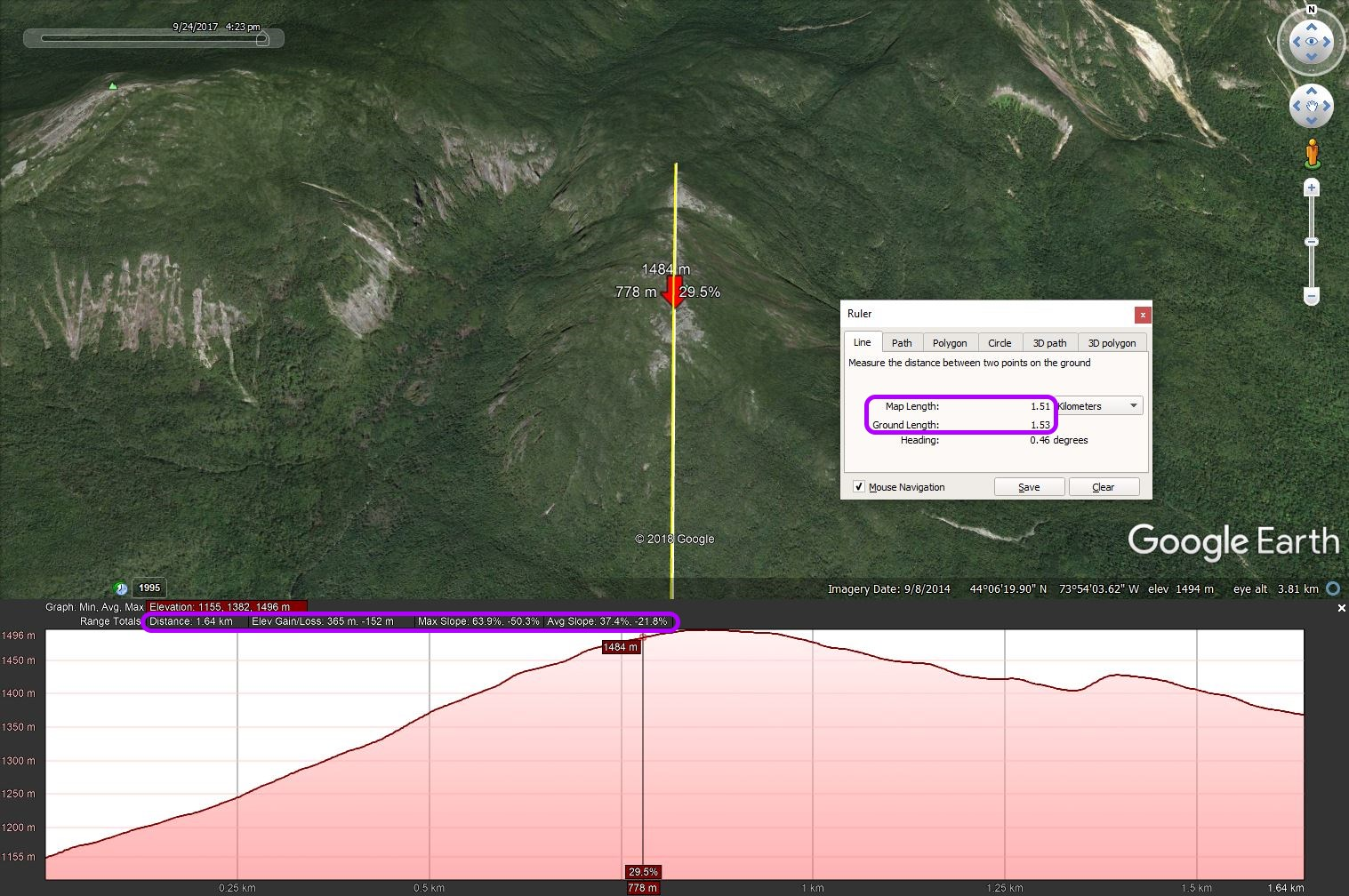Distance to the surface of the mountain.

Maxim shared this idea ago
Declined

The track section contains the distance on the horizontal plane. For traveling in the mountains I want to see the distance traveled along the surface of the mountain. Few programs can do this. It will be good for climbers and tourists in the mountains (hiking).

Replies (7)1

Good day Maxim,

impressive track record, congratulation!

Even in this case of huge elevation changes, final distance won't be so different compare to flat value visible in Locus. From where comes your "Surface value"? Because if I compute correctly, in your case additional distance should be little more then 1 km and not almost 15 kilometres! If your value is correct, then it should make sense to display such value anyway if my value is correct, then I'm not sure if it worth it to use space for display of almost identical (1% more) value.1

On the pictures of the number for example, so you understand what I'm talking about. In my case, the distance the surface is completely different.1

Oki,

as I wrote ... may you please share with me your track? I have some own tracks recordings from Alps, but not of them are so long and with such dynamic elevation changes. If you do not want to share it in public, send it on my email jiri.mlavec@asamm.com , thank you!1

Mathematical calculation:

c=100,3 km

v=1,7 km

a+b=100,358 km

difference=0,058 km (58m) 0.06%

Of course, this model does not have a terrain relief!2

@Maxim

I agree with Menion that "surface distance" is typically negligibly larger than horizontal distance. What did you use to calculate a "surface distance" that's ~15% greater?

Calculating "surface distance" is a problem involving fractals. In other words, it depends on how much detail you are considering; a smaller "measuring stick" will record a larger amount.

For example, if you measure the trail's distance using a measuring wheel with a circumference of two meters, it will produce a different result than a wheel with a circumference of 50 cm. Why? Because the smaller wheel can follow along on smaller irregularities in the trail (up and over and around rocks, bumps, and holes) compared to the larger wheel which just glides over the irregularities. The classic example of this problem is measuring the length of a coastline (like the coastline of Norway). The coastline is much longer if you include every cove, bay, and fjord.

In addition, if you were to calculate the "surface distance" using a DEM (Digital Elevation Model, like SRTM) you will definitely get a different result compared to a measuring wheel. The DEM is a mathematical model based on measurements made at (typically) 100 meter intervals. That means all points between the measured intervals are interpolated (mathematical estimations).1

Of course. I understand it.

I can imagine a model of calculation for every 100m.

It is questionable how much computing power it would consume.

A solution with a larger scale (1000m) would lose its significance.

I think the estimate for the route chart, for example, +10% per 1000m high, will give a rough result, as in the case of complex heavy calculations.

Only the hiking alone shows the actual terrain.

It would help us mathematically the "exact" number?1

Thanks Taras for a quite precise analyze. Necessary computing power is here close to zero, this is not a problem.

Maxim, if you upload here your track, I may compute surface distance in Locus, so we may talk about precise numbers for your record. Anyway I'm still a lot closer to conviction, that additional "surface distance" will be too close to current value for major of users => confusing (and useless).1

Another my track.

This track in the Google Earth program.

When the accuracy is above the difference in distances will be greater. If the angle of inclination is greater, the difference in distances is larger, too. I think it's not difficult to show this value. You can turn this value on or off in the settings.2

Maxim,

In Unnamed.jpg (Безымянный.jpg), did you manually draw the measurements (shown in green) on Google Earth's Elevation Profile?

I am not an expert with Google Earth Pro but I cannot find a feature to automatically draw what is shown in the image. So I have to assume you drew it manually.

• Map Distance: flat; excludes elevation.
• Ground Distance: 3D; includes elevation.

Here is an example showing the computed Map and Ground Distances for a steep route (maximum slope is ~64% and the average slope is 37%). The difference between Map and Ground Distances is 20 meters (1.51 km vs 1.53 km).1

Because of the minor benefit that this feature brings, because of minor difference between horizontal and 3D distance and also minor interest, I'm closing this idea.

Menion